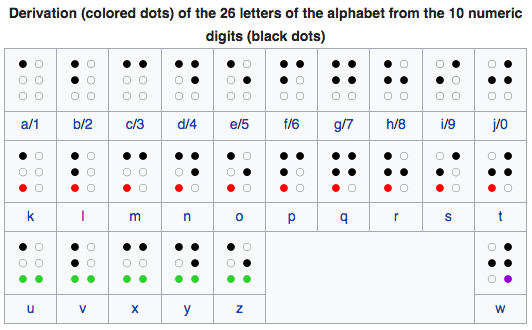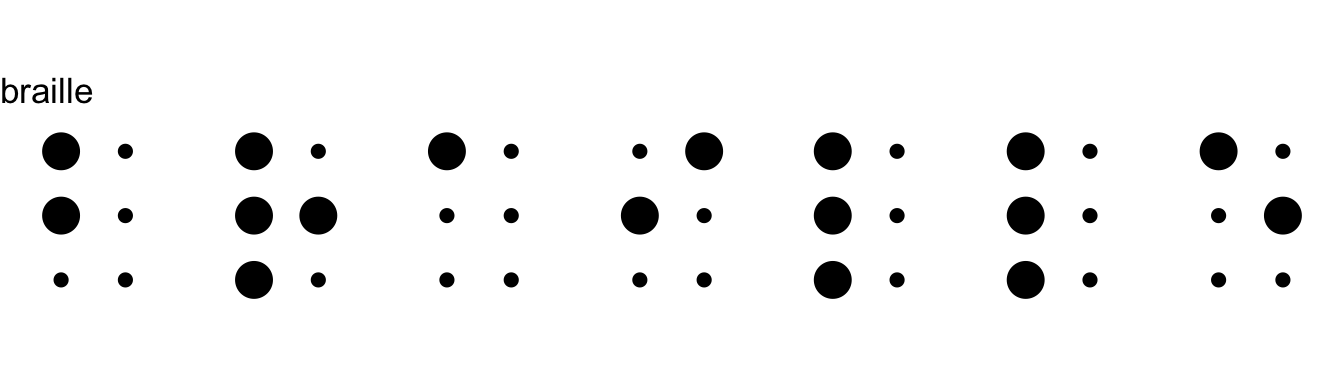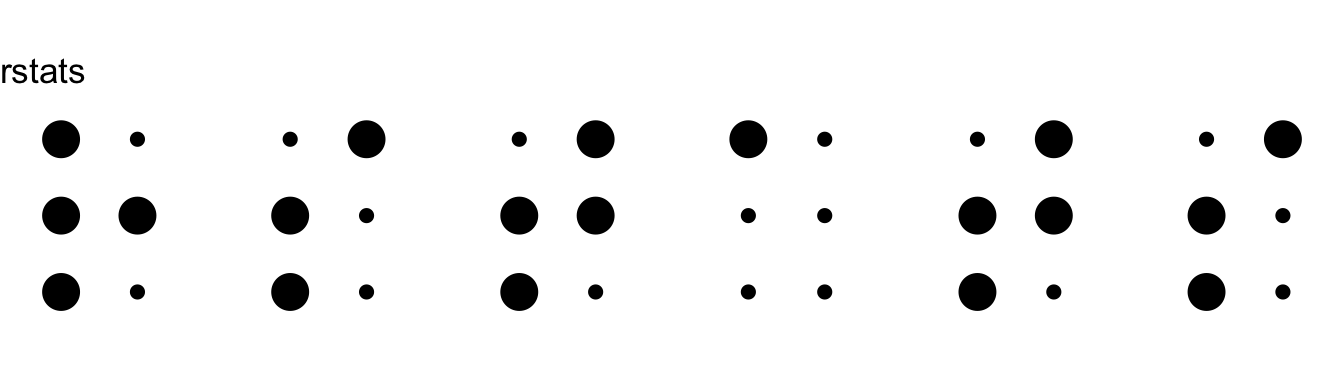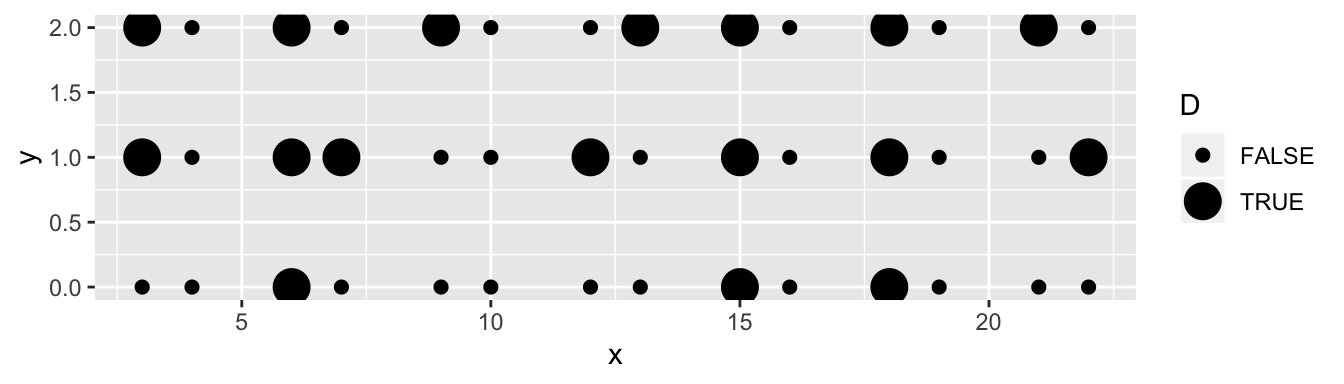# Encoding and Rendering Grade 1 Braille

## Introduction

Braille is a tactile writing system used by people who are visually impaired.

A pattern of 2x3 dots is used to encode symbols, letters, words and language. The current(?) standard(?) for the English language is Unified English Braille, although Nemeth Braille seems to be a slightly different standard which still appears popular for encoding mathematical notion.

Disclaimer:

• I’m only looking at simple Grade 1 encoding (i.e. letter-by-letter transcription)
• I realise there are Braille symbols in unicode, but I’m thinking beyond screen/paper representations, so I want to render my own symbols.

## Braille subset - just the lowercase letters## Defining a Braille symbol

• A Braille symbol is made up of 6 dots.
• Therefore, we need only 6 bits to encode it
• Let’s round it up to 8 bits, and store the definition for each symbol in a byte.
• Assign each of the dots a numerical value.
• The numerical representation of a symbol is the sum of its dots
• e.g.
• the letter `g` is represented by setting the upper 4 dots
• sum of upper 4 dots = 1 + 2 + 4 + 8 = 15

To turn a symbol back into a sequence of ON/OFF values, use `intToBits` e.g.

``````dots <- intToBits(15)[1:6]
matrix(dots, ncol=2, byrow=TRUE)``````
``````     [,1] [,2]
[1,]   01   01
[2,]   01   01
[3,]   00   00``````

## Exploiting redundancy

In the table of all the alphabet Braille symbols in the image above, you can see that the second set of 10 symbols is just a copy of the first set with the bottom left dot turned on.

Similarly the third set of 10 symbols is a copy of the first set but with both bottom dots turned on.

So for my current purposes, it’s only necessary to carefully define the first row, and then just calculate all other rows from it.

## The alphabet

• Create the first 10 letters of the alphabet by summing the dots in each.
• Create subsequent rows by adding the extra dots that are set for that row.
``````set1 <- c(1, 5, 3, 11, 9, 7, 15, 13, 6, 14)
set2 <- set1 + 16
set3 <- set1 + 48
set4 <- set1 + 32

# Definition of the first 40 symbols
symbols <- c(set1, set2, set3, set4)

symbols``````
``````   1  5  3 11  9  7 15 13  6 14 17 21 19 27 25 23 31 29 22 30 49 53 51 59 57
 55 63 61 54 62 33 37 35 43 41 39 47 45 38 46``````

## Lookup table

For historical reasons, the letter `w` is totally out of sequence, so the mapping from letter to symbol is a bit weird.

``````letter_to_symbol_idx <- setNames(1:26,letters)
letter_to_symbol_idx['w'] <- 40
letter_to_symbol_idx['x'] <- 23
letter_to_symbol_idx['y'] <- 24
letter_to_symbol_idx['z'] <- 25

letter_to_symbol_idx``````
`````` a  b  c  d  e  f  g  h  i  j  k  l  m  n  o  p  q  r  s  t  u  v  w  x  y  z
1  2  3  4  5  6  7  8  9 10 11 12 13 14 15 16 17 18 19 20 21 22 40 23 24 25 ``````

## Simple Braille Renderer (Grade 1)

Split a word into characters and find all the symbols for these characters

``````this_word     <- "braille"
chars         <- strsplit(tolower(this_word),'')[]
symbol_idx    <- letter_to_symbol_idx[chars]
these_symbols <- symbols[symbol_idx]

these_symbols``````
``  5 29  1  6 21 21  9``

Turn the symbols into ON/OFF dot definitions

``````dots <- these_symbols %>% map(~intToBits(.x)[1:6] > 0)
dots``````
``````[]
  TRUE FALSE  TRUE FALSE FALSE FALSE

[]
  TRUE FALSE  TRUE  TRUE  TRUE FALSE

[]
  TRUE FALSE FALSE FALSE FALSE FALSE

[]
 FALSE  TRUE  TRUE FALSE FALSE FALSE

[]
  TRUE FALSE  TRUE FALSE  TRUE FALSE

[]
  TRUE FALSE  TRUE FALSE  TRUE FALSE

[]
  TRUE FALSE FALSE  TRUE FALSE FALSE``````

## Create a data frame of dots positions

``````# Define x and y positions of each dot
x <- c(0, 1, 0, 1, 0, 1)
y <- c(2, 2, 1, 1, 0, 0)

# merge each dot with its (x, y) coordiante,
# and add some spacing to the x cordinate so that
# the characters appear one after the other
plot_df <- data.frame(
dot = unlist(dots),
x   = x + rep(seq(dots), e=6) * 3,
y   = y
)

dot x y
TRUE 3 2
FALSE 4 2
TRUE 3 1
FALSE 4 1
FALSE 3 0
FALSE 4 0

## `ggplot`

``````# Simple plot
ggplot(plot_df) +
geom_point(aes(x, y, size=dot)) +
coord_equal() +
theme_void() +
ggtitle(this_word) +
theme(legend.position = 'none') +
expand_limits(y=c(-0.5, 2.5))``````## Tweetable Simple Braille Renderer (Grade 1)

``````#rstats
library(tidyverse)
d=c(1,5,3,11,9,7,15,13,6,14)
d=c(d,d+16,d+48,d+32)
L=setNames(c(1:22,40,23:25),letters)
D=map(d[L[strsplit('braille','')[]]],~intToBits(.x)[1:6]>0)
P=data.frame(D=unlist(D),x=0:1+rep(seq(D),e=6)*3,y=rep(2:0,e=2))
ggplot(P)+geom_point(aes(x,y,size=D))``````
``Warning: Using size for a discrete variable is not advised.``